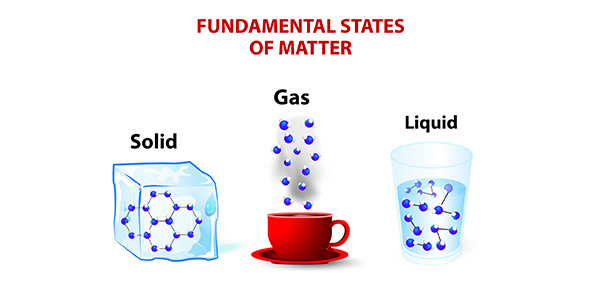# Matter Period 4

41 QuestionsSettingsUnit Assessment on Structure of Matter

Related Topics
• 1.
All matter is made up of atoms or molecules?  True or False
• A.

True

• B.

False

• 2.
Are atoms mostly full or mostly empty space?
• A.

Mostly Full

• B.

Mostly Empty Space

• 3.
What makes the atoms of one element different from the atoms of another element?
• A.

The number of protons in the nucleus

• B.

The number of electrons in the outer shell

• C.

The number of neutrons in the nucleus

• D.

The average mass of the isotopes

• 4.
How many naturally occuring elements are found?
• A.

1

• B.

15

• C.

92

• D.

118

• 5.
A molecule does not need more than one element to be considered a "compound".   True or False
• A.

True

• B.

False

• 6.
It is possible to have a molecule that is not a compound...True or False
• A.

True

• B.

False

• 7.
All compounds are molecules....True or False
• A.

True

• B.

False

• 8.
Is O2 a molecule?
• A.

YES

• B.

NO

• 9.
Is O2 a compound?
• A.

YES

• B.

NO

• 10.
How many compounds are in the following image?
• A.

2

• B.

6

• C.

19

• D.

4

• 11.
How many molecules are in the following image?
• A.

2

• B.

6

• C.

19

• D.

4

• 12.
Is air a mixture or a pure substance?
• A.

Mixture

• B.

Pure substance

• 13.
Is baking soda (NaHCO3) a mixture or a pure substance?
• A.

Mixture

• B.

Pure substance

• 14.
Is 100% pure orange juice a mixture or a pure substance?
• A.

Mixture

• B.

Pure substance

• 15.
Is blood a heterogeneous or a homogenous mixture?
• A.

Homogeneous

• B.

Heterogeneous

• 16.
Is a oil and water mixture heterogeneous or a homogenous mixture?
• A.

Homogeneous

• B.

Heterogeneous

• 17.
What is it called when multiple pure substances are added together?
• A.

Compound

• B.

Element

• C.

Mixture

• D.

Molecule

• 18.
What are the only two types of pure substances?
• A.

Elements and compounds

• B.

Elements and atoms

• C.

Atoms and molecules

• D.

Heterogeneous and homogeneous

• 19.
Most of the hot flavor in peppers comes from capsaicin, C18H27NO3, Capsaicin is a(n)
• A.

Element

• B.

Pure substance

• C.

Diatomic molecule

• D.

Mixture

• 20.
Changing the amount of energy or pressure will affect the states of matter
• A.

True

• B.

False

• 21.
Which of the following is not a state of matter?
• A.

Solid

• B.

Gas

• C.

Condesate

• D.

Liquid

• 22.
What is the following state of matter?  the particles are spread very far apart and moving at high rates of speed
• A.

Solid

• B.

Liquid

• C.

Gas

• 23.
The particles in a liquid and gas are always in motion but the particles in a solid stop moving?  T/F
• A.

True

• B.

False

• 24.
When a substances goes from a solid to a liquid it is called….
• A.

Melting

• B.

Deposition

• C.

Sublimation

• D.

Freezing

• 25.
When a substance goes from a gas to a solid it is called…
• A.

Melting

• B.

Deposition

• C.

Sublimation

• D.

Freezing

• 26.
What is dry ice going from a solid directly to a gas an example of?
• A.

Melting

• B.

Deposition

• C.

Sublimation

• D.

Freezing

• 27.
Water found on the outside of a glass is called what?
• A.

Melting

• B.

Vaproization

• C.

Condensation

• D.

Freezing

• 28.
Do chemical or physical changes involve chaniging the states of matter (solid, liquid, gas)?
• A.

Chemical

• B.

Physical

• 29.
When a substance changes from a liquid to a gas it loses mass.  T/F
• A.

True

• B.

False

• 30.
Is energy gained or lost during sublimation?
• A.

Gained

• B.

Lost

• 31.
When energy is added to a solid substance what happens to the particles?
• A.

The do not change

• B.

They speed up

• C.

They slow down

• D.

They combine

• 32.
Where could you find information about the flammability of a product?
• A.

MSDA

• B.

MSDS Sheet

• C.

A lab report

• D.

• 33.
Is the oxidation of a substance a chemical or physical property?
• A.

Chemical

• B.

Physical

• 34.
Do chemical or physical properties involve forming a new substance?
• A.

Chemical

• B.

Physical

• 35.
Cooking an egg is an example of a....
• A.

Chemical change

• B.

Physical change

• 36.
photosynthesis is an example of a....
• A.

Chemical change

• B.

Physical change

• 37.
Mixing together salt and water is an example of a....
• A.

Chemical change

• B.

Physical change

• 38.
Which section represent water in the liquid form only?
• A.

Section 1

• B.

Section 2

• C.

Section 3

• D.

Section 4

• 39.
Which container ha sthe liquid that is the LEAST dense?
• A.

X

• B.

Y

• C.

W

• D.

Z

• 40.
What is the emperical formula?
• A.

CCl2H2

• B.

C2ClH

• C.

CCLh7

• D.

C3L2H2

• 41.
Is Aluminum (Al) a mixture or a pure substance?
• A.

Mixture

• B.

Pure substance